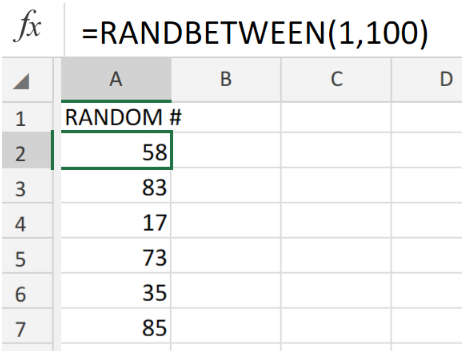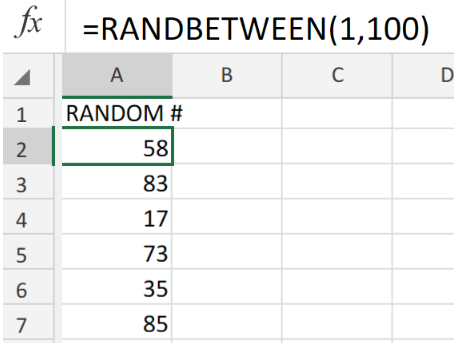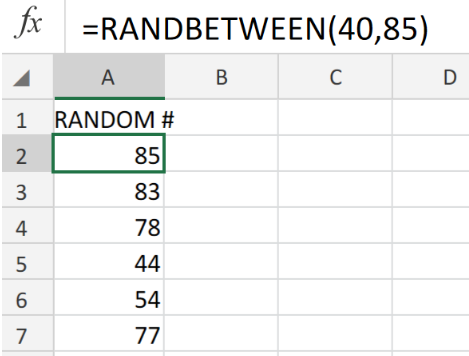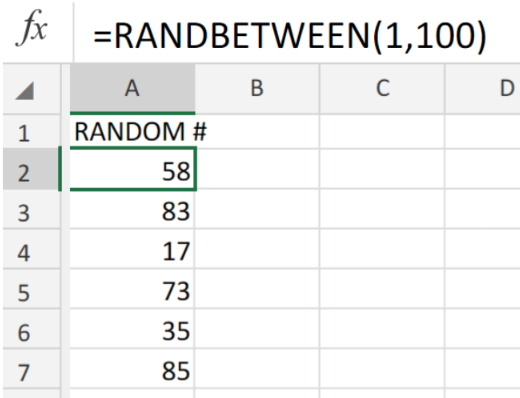Get instant live expert help with Excel or Google Sheets“My Excelchat expert helped me in less than 20 minutes, saving me what would have been 5 hours of work!”

#### Post your problem and you’ll get expert help in seconds.

Your message must be at least 40 characters
Our professional experts are available now. Your privacy is guaranteed.

# Random number between two numbersFigure 1. of the Randbetween Function in Excel.

The Excel Randbetween Function determines a random  number integer between the number range we have specified. This tutorial will step through the process of generating random numbers that fall between two specified values

## Generic Formula

`=RANDBETWEEN(lower,upper)`

The Randbetween operation syntax consists of the following arguments;

• Lower (required) – The smallest number integer within the range we have specified.
• Upper (required) – The largest number integer within the range we have specified.

## How to use the Randbetween Function in Excel.

In the event that we are required to generate random number integers between 1-100, follow these simple steps;

1. Select any cell in our Excel sheet. In the example illustrated below we have selected cell A1.
2. In cell A2, insert the following formula `=RANDBETWEEN(1,100)`Figure 2. of the Randbetween Function in Excel.

1. Copy the operation syntax down the entire cells in the column for more results (as illustrated above)

In the event that we are required to generate random number integers between (40-85), follow these simple steps;

1. Select any cell in our Excel sheet. In the example illustrated below we have selected cell A1.
2. Input the formula syntax `=RANDBETWEEN(40,85) `into the formula bar for cell A1, then press Enter.Figure 3. of the Randbetween Function in Excel.

1. Copy the operation syntax down the entire cells in the column for more results (as illustrated above)

## Note

The Excel RANDBETWEEN function will by default, generate new random number integers whenever we effect a change on our worksheet. This includes any editing made on our worksheet, also also when we are simply opening our worksheet.Figure 4. of Final Result

## Instant Connection to an Expert through our Excelchat Service

Our live Excelchat Service is here for you. We have Excel Experts available 24/7 to answer any Excel questions you may have. Guaranteed connection within 30 seconds and a customized solution for you within 20 minutes

### Did this post not answer your question? Get a solution from connecting with the expert.Another blog reader asked this question today on Excelchat:
Related blogs
Solution examplesI'm Apply a =RANDBETWEEN formula in one cells want to apply Formula to entire column and run formula when i hit the entire on the particular cell
Solved by M. S. in 20 minsHelp! In a coin toss I have a 1 in 2 (fifty-fifty) chance of getting heads or tails. I need a formula to determine my chance of getting 4 heads in a row or 4 tails in a row out of 10 coin tosses. If I get 4 in a row I win. What formula can I use to determine my chances of winning if I repeat the 10 tosses for hundreds or thousands of times? I get as far as =RANDBETWEEN(0,1) but I have not been able to figure out what formula to use to determine if there are 4 in a row in a group of 10 tosses, times thousands of groups with 10 tosses in each group. Thank-you for any help you can provide.
Solved by V. F. in 51 minsI have 4 column. when i fill the first 3 column. in 4th column i need a unique id number and i generate unique id number using RANDBETWEEN formula [ =CHAR(RANDBETWEEN(65,90))&CHAR(RANDBETWEEN(65,90))&RANDBETWEEN(1000,9900) ] but i want ..... when i'm filling the 3 column after that 4th column run this formula and generate unique id automatically
Solved by X. Q. in 20 minswhen i fill column A and B then C column generate Unique ID using RandBetween Formula {=CHAR(RANDBETWEEN(65,90))&CHAR(RANDBETWEEN(65,90))&RANDBETWEEN(1000,9900)} ...
Solved by S. Q. in 20 mins## Subscribe to Excelchat.coAnother blog reader asked this question today on Excelchat: